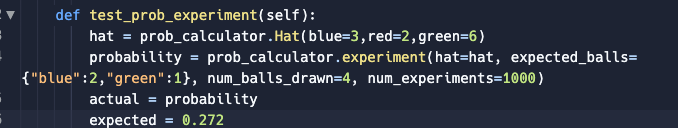# Python Certificate - probability Calculator wrong Test values

Hello Everyone,
i am currently working on the probability Calculator for the Scientific Programming python Certificate. And i cant get the last test (“test_prob_experiment”) running, as i dont cant get the correct Value for the expected probability. Can it be the case that the wished value is not correct set ? Because every solution i’ve compared ran into the same problemI would like to but i’m not sure wether i would violate the rules for the forum as this is a Certificate Task. But i guess if the value is correct I need to find the error. Thank you for your fast reply!

You can post your code and ask questions on the forum - that’s what the forum is for.

Okay there you go``````import copy
import random

# Consider using the modules imported above.

class Hat:

def __init__(self, **kwargs):
self.contents = []
for name, number in kwargs.items():
for j in range(0, int(number)):
self.contents.append(name)

def draw(self, number_of_drawables):
draws = []
if number_of_drawables > len(self.contents):
return self.contents

for ball in range(0, number_of_drawables):
ball = random.choice(self.contents)
draws.append(ball)
self.contents.remove(ball)
return draws

def experiment(hat, expected_balls, num_balls_drawn, num_experiments):

def check_expectations(actuals, expected):

draws = {}
for i in actuals:
if i in draws:
draws[i] = draws[i] + 1
else:
draws[i] = 1
actuals = draws

for color,count in expected.items():
if color not in actuals:
return False
elif count>actuals[color]:
return False
else:
return True

draws = {}
for i in actuals:
if i in draws:
draws[i] = draws[i] + 1
else:
draws[i] = 1
actuals = draws
return actuals

success = 0
for i in range(0, num_experiments):

try:
copied_hat = copy.deepcopy(hat)
except copy.Error as e:
print(f'An error Occured while copying object {e}')

actuals = copied_hat.draw(number_of_drawables=num_balls_drawn)
if check_expectations(actuals, expected_balls):
success = success + 1

return success / num_experiments

``````

Why this condition?

to prevent a wrong value if there are not enough balls in the correct color. E.g if you are expecting two yellow balls, but only one is drawn

You are always returning false with that condition somehow, so its not doing what you think

What do you mean exactly ?

AssertionError: 0.0 != 0.272 within 0.01 delta (0.272 difference) : Expected experiment method to return a different probability.

Did you see this error?

I found one bug that is contributing to this -

This line is indented wrong

When I replace this with my code for the same, it works, so the error is in this function here.

Oh yeah this was an indention error. I must have made a mistake while copying. I get 0.293 as resulting probability. But let me double check the function

Ahha, error is here

1 Like

I think i still dont understand the error. Can you maybe explain ? Im feeling kinda lostGosh found it. The else was unnecessary and wrong! Now it works like a charme! Thank you for your support!

1 Like